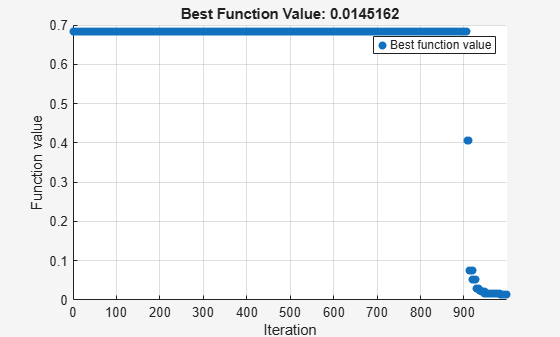# Optimize Multidimensional Function Using `surrogateopt`, Problem-Based

This example shows how to minimize a multidimensional function using surrogate optimization in the problem-based approach. The function to minimize, `multirosenbrock(x)`, appears at the end of this example. The `multirosenbrock` function has a single local minimum of `0` at the point `[1,1,...,1]`. The function is designed to be challenging for solvers to minimize.

Note: The code for the `multirosenbrock` helper function is provided at the end of this example. Make sure the code is included at the end of your script or in a file on the path.

Create a 4-D optimization variable `x`. The `multirosenbrock` function expects the variable to be a row vector, so specify `x` as a 4-element row vector.

`x = optimvar("x",1,4);`

The `surrogateopt` solver requires finite bounds on all problem variables. Specify lower bounds of –3 and upper bounds of 3. When you specify scalar bounds, they apply to all problem variables.

```x.LowerBound = -3; x.UpperBound = 3;```

To use `multirosenbrock` as the objective function, convert the function to an optimization expression using `fcn2optimexpr`.

`fun = fcn2optimexpr(@multirosenbrock,x);`

Create an optimization problem with the objective function `multirosenbrock`.

`prob = optimproblem("Objective",fun);`

Solve the problem, specifying the `surrogateopt` solver.

```rng default % For reproducibility [sol,fval] = solve(prob,"Solver","surrogateopt")```
```Solving problem using surrogateopt. ``````surrogateopt stopped because it exceeded the function evaluation limit set by 'options.MaxFunctionEvaluations'. ```
```sol = struct with fields: x: [0.1017 0.0169 0.3921 0.1585] ```
```fval = 1.1830 ```

### Attempt to Improve Solution

The returned solution is not good, because the objective function value is not very close to `0`. Try to improve the solution by running `surrogateopt` for more evaluations. Use the previous solution as a start point.

```options = optimoptions("surrogateopt","MaxFunctionEvaluations",1000); [sol2,fval2] = solve(prob,sol,"Solver","surrogateopt","Options",options)```
```Solving problem using surrogateopt. ``````surrogateopt stopped because it exceeded the function evaluation limit set by 'options.MaxFunctionEvaluations'. ```
```sol2 = struct with fields: x: [0.9212 0.8487 0.8199 0.6725] ```
```fval2 = 0.0387 ```

This time, the solver reaches a good solution.

### Helper Function

This code creates the `multirosenbrock` helper function.

```function F = multirosenbrock(x) % This function is a multidimensional generalization of Rosenbrock's % function. It operates in a vectorized manner, assuming that x is a matrix % whose rows are the individuals. % Copyright 2014 by The MathWorks, Inc. N = size(x,2); % assumes x is a row vector or 2-D matrix if mod(N,2) % if N is odd error('Input rows must have an even number of elements') end odds = 1:2:N-1; evens = 2:2:N; F = zeros(size(x)); F(:,odds) = 1-x(:,odds); F(:,evens) = 10*(x(:,evens)-x(:,odds).^2); F = sum(F.^2,2); end```